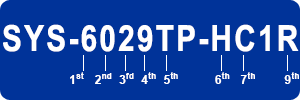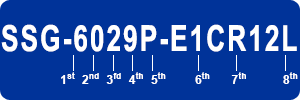#Product Naming Convention - SuperServer® with 3.5" HDDsCharacter Representation Options 1st CPU • 5 = Single processor         • 6 = Dual processor         • 7 = Dual processor (Tower) 2nd Reserved • 0 = Reserved 3rd Form Factor • 1 = 1U         • 2 = 2U         • 3 = 3U         • 4 = 4U 4th Generation • 9 = X11 Series         • 8 = X10 Series         • 7 = X9 Series         • 6 = X8 Series         • 5 = X7 Series 5th Chipset / System Platform • P = Socket P (X11) / Panther Point (X7)         • K = Xeon Phi / Socket P         • GP = GPU/Coprocessor (X11)         • GQ = Quad GPGPU         • GR = GPGPU         • A = Workstation         • B = Bigby / Socket B2         • C = Cougar Point         • D = Denlow platform         • I = Foxhollow         • M = Mukilteo         • R = Socket R         • S = Skylake         • T = Tylersburg         • U = Ultra         • UX = Ultra Hyper-Speed         • AX = Overclocking 6th Interface / Other • 3 = SAS (SCU) (X8)         • 6 = SAS2 (X8)         • 70 = LSI 2008         • 71 = LSI 2108         • 72 = LSI 2208         • 73 = LSI 2308         • C0 =SAS3 IT Mode         • C1 = SAS3 HW RAID         • D = Data Center Optimized (X10) / Desktop (X8)         • E = Extension Slot with Riser         • E1C = Single SAS3 Expander         • H = Hot Swap (X8) / Support x16 device (GPGPU)         • I = Internal Drives         • M = Mini-Chassis         • N = NVMe (X10, X11)         • N# = # of NVMe drives (X10, X11)         • N3 = SAS without UIO (X8)         • NT = SATA without UIO (X8)         • P = Internal slot / Passive         • T = SATA         • U = UIO (X8)         • W = WIO         • X = MaxIO (Socket R) / NVLink (GPGPU) 7th Power Type • R = Redundant Power Supplies 8th Connectivity • + = 18 DIMM (X8) / 24 DIMM (X9,X10) / FPGA (X11)         • 25M = 25GbE Mellanox         • 2 = 2 LAN ports         • 4 = 4 LAN ports         • 8 = 8 Internal Drives         • F = IPMI (onboard BMC) (X7, X8)         • -NF# = Xeon Phi 72## Processor         • P = SFP+         • T = 10GbE

#Product Naming Convention - Twin/TwinPro/BigTwin (3.5" HDD)Character Representation Options 1st CPU • 6 = Dual processor 2nd Reserved • 0 = Reserved 3rd Form Factor • 1 = 1U         • 2 = 2U 4th Generation • 9 = X11 Series         • 8 = X10 Series         • 7 = X9 Series 5th Chipset / System Platform • BT = BigTwin         • TP = TwinPro (X10, X11)         • PR = TwinPro (X9)         • TK = TwinPro (Xeon Phi)         • TR = Twin 6th # of Nodes • D: 2 nodes         • H: 4 nodes 7th Interface / Other • 70 = Broadcom 2008         • 71 = Broadcom 2108         • 72 = Broadcom 2208         • 73 = Broadcom 2308         • C0 = SAS3 IT Mode, Broadcom 3008         • C1 = SAS3 HW RAID, Broadcom 3108         • N = NVMe         • T = SATA 8th Connectivity • F: IB FDR / 40G Ethernet         • Q: QDR         • T: 10GBase-T 9th Power Type • R = Redundant Power Supplies 10th Others • + = 24 DIMM (X10) / 16 DIMM (older X9)         • F = Onboard BMC (older X9)         • -SIOM = SIOM slot (X10)

#Product Naming Convention - FatTwin (3.5" HDD)Character Representation Options 1st Product Series • F = FatTwin 2nd Number of processor(s) • 5 = UP         • 6 = DP         • 8 = QP 3rd Tray Height /Form Factor • 1 = 1U         • 2 = 2U         • 4 = 4U 4th System Generation • 9 = X11 Series         • 8 = X10 Series         • 7 = X9 Series 5th Chipset / Socket / Platform • P = Socket P         • R = Socket R         • B = Socket B2         • G = GPU node         • H = Hadoop node 6th HDD/Tray Form Factor • 3: Half width tray with 3.5" drives         • 6: Full width tray with 3.5" drives 7th I/O Direction • F = Front I/O         • R = Rear I/O 8th Controller Interface • 72: Broadcom 2208 controller         • 73: Broadcom 2308 controller         • T: Intel SATA         • C0: Broadcom 3008 controller         • C1: Broadcom 3108 controller 9th and 10th Additional • B = Rear HDDs         • PT = 10G LAN         • + = 16 DIMMs (X9, X10)         • L = Cost effective         • N = NVMe drives         • F = Infiniband 56Gbps

#Product Naming Convention - SuperStorage (3.5" HDD)Character Representation Options 1st CPU • 5 = UP         • 6 = DP         • 7 = DP (Tower) 2nd Reserved • 0 = Reserved 3rd Form Factor • 1 = 1U         • 2 = 2U         • 3 = 3U         • 4 = 4U 4th System Generation • 9 = X11 Series         • 8 = X10 Series         • 7 = X9 Series 5th Socket / Family • P = Socket P         • R = Socket R         • D2 = Socket F / 2-Core         • D4 = Socket F / 4-Core         • D8 = Socket F / 8-Core         • T = Tylersberg         • A = Atom SoC 6th Interface Type • A: Direct Attached         • AC: Direct Attached SAS3         • D: Dual Motherboard         • DC: Dual Motherboard SAS3         • E1: Single Expander         • E1C: Single SAS3 Expander         • E2: Dual Expander         • E2C: Dual SAS3 Expander         • N: NVMe 7th Power Redundancy • R = Redundant Power Supplies 8th Additional • ##: Number of drive bays         • 4: 4 LAN ports         • L: IT Mode         • H: Hardware RAID         • N: Hardware RAID & 24 DIMMs (X9/X10) /           NVMe (X10 Simply Double)         • NV: NVDIMM         • T: HW RAID up to 16 drives